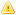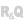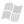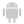Home |Sign in | EnglishHelpEditorsControls (window/page/report)ControlsSpreadsheet controlThe Spreadsheet control: Available formulasOverviewFormulas available in standardSee alsoEvents associated with a Spreadsheet controlHandling a Spreadsheet control by programming (in a report)Handling a Spreadsheet control by programming (in a window)Handling a Spreadsheet control by programming (in a window) (prefixed syntax)Printing the content of a Spreadsheet control (AAF)Properties associated with the Spreadsheet controlSpreadsheet control in a reportSpreadsheet control in a windowSpreadsheet: Using the ribbonThe Spreadsheet controlThe Spreadsheet control: Available formulasThis content has been translated automatically. Click here to view the French version.
• Overview
• Formulas available in standard
WINDEVWEBDEVWINDEV MobileOthersOverview
At run time, in the Spreadsheet control, the end user directly enters the calculation formulas. The cells used can be selected with the mouse.
The Spreadsheet control proposes the main calculation functions and operators: sum, subtraction, multiplication, division, percentages, mean, min-max, condition (IF), calculation and comparison operators, ...
Several WLanguage functions can be used in the formulas such as the mathematical functions, string functions, date functions, financial functions, ...
In the formulas, you have the ability to use the WLanguage procedures that have been created for this purpose in the application (SpreadsheetAllowProcedure). For example, the application can propose the "Shipping cost" procedure that calculates the amount according to advanced parameters: weight, volume, speed, destination country, ...
This procedure can even be used in the spreadsheet!
Formulas available in standard
The available functions are as follows:
 Compatibility functions COVAR Returns the covariance, mean deviation of products for each series of observations. MODE Returns the most common value of a data series. STDEV Evaluates the standard deviation of a population according to a sample of this population . VAR Calculates the variance according to a sample. Date and time functions DATE Returns the series number for a specific date. DATEVALUE Converts a date represented in text format into serial number. DAY Converts a series number into a day of the month. DAYS360 Returns the number of days between two dates according to a 360-day year. EDATE Returns the series number of the date that represents a specific date (start_date argument), corrected plus or minus the specified number of months. EOMONTH Returns the series number of the last day of the month before or after a specified number of months from start_date. HOUR Converts a series number into hour (included between 1 and 12). MINUTE Converts a series number into minute (included between 0 and 59). MONTH Converts a series number into month (included between 1 and 12). NETWORKDAYS Returns the number of whole workdays included between two dates. NETWORKDAYS.INTL Returns the number of whole workdays between two dates via parameters identifying the weekend days and their number. NOW Returns the series number of today's date and time. SECOND Coverts a series number into second (between 0 and 59). TIME Returns the series number of a specific time. TIMEVALUE Converts a date represented in text format into serial number. TODAY Returns the series number of today's date. WEEKDAY Converts a series number into a day of the week (included between 0 and 7). WEEK.NUM Converts a series number into a number representing the week number in the year. WORKDAY Returns the series number of the date before or after the specified number of days. WORKDAY.INTL Returns the series number of the date before or after the specified number of days by specifying the parameters that identify and count the days included in the weekend. YEAR Converts a series number into year. YEARFRAC Returns the fraction of the year representing the number of whole days separating the start date from the end date. Engineering functions CONVERT Converts a number from a measurement unit to another one. DEC2BIN Converts a decimal number into binary number. DEC2HEX Converts a decimal number into hexadecimal number. GESTEP Checks whether a number is greater than a threshold value. HEX2BIN Converts an hexadecimal number into binary number. HEX2DEC Converts an hexadecimal number into decimal number. HEX2OCT Converts an hexadecimal number into octal number. OCT2BIN Converts an octal number into binary number. OCT2DEC Converts an octal number into decimal number. OCT2HEX Converts an octal number into hexadecimal number. Information functions CELL Returns information about the layout, the location and the content of a cell. NOTE This function is not available in Excel Web App. ISBLANK Returns TRUE if the value argument is empty. ISERR Returns TRUE if the value argument refers to an error value, except #N/A. ISERROR Returns TRUE if the value argument refers to an error value. ISEVEN Returns TRUE if the digit is even. ISLOGICAL Returns TRUE if the value argument refers to a logical value. ISNA Returns TRUE if the value argument refers to the #N/A error value. ISNONTEXT Returns TRUE if the value argument is not presented in text format. ISNUMBER Returns TRUE if the value argument represents a number. ISODD Returns TRUE if the digit is odd. ISREF Returns TRUE if the value argument is a reference. ISTEXT Returns TRUE if the value argument is presented in text format. N Returns a value converted into number. NA Returns the #N/A error value. TYPE Returns a number indicating the data type of a value. Logical functions AND Returns TRUE if all its arguments are TRUE. FALSE Returns the logical value FALSE. IF Specifies a logical test to run. IFERROR Returns a specific value if a formula generates an error ; otherwise, returns the result of the formula. NOT OR Returns TRUE if one of the arguments if TRUE. TRUE Returns the logical value TRUE. Search and reference functions ADDRESS Returns a reference in text format to a single cell of a worksheet. AREAS Returns the number of areas in a reference. CHOOSE Chooses a value in a list. COLUMN Returns the column number of a reference. COLUMNS Returns the number of columns in a reference. HLOOKUP Performs a search in the first row of a matrix and returns the value of the specified cell. INDIRECT Returns a reference indicated by a text value. LOOKUP Searches for values in a vector or in a matrix. MATCH Searches for values in a reference or in a matrix. OFFSET Returns a reference shifted in relation to a given reference. ROW Returns the row number of a reference. ROWS Returns the number of rows in a reference. TRANSPOSE Returns the transposition of a matrix. VLOOKUP Performs a search in the first column of a matrix and moves on the row to return the value of a cell. Mathematical and trigonometric functions ABS Returns the absolute value of a number. ACOS Returns the arc cosine of a number. ACOSH Returns the reverse hyperbolic cosine of a number. AGGREGATE Returns an aggregate in a list or database. ASIN Returns the arc sine of a number. ASINH Returns the reverse hyperbolic sine of a number. ATAN Returns the arc tangent of a number. ATAN2 Returns the arc tangent of the x and y coordinates. ATANH Returns the reverse hyperbolic tangent of a number. CEILING Rounds a number to the nearest integer number or to the nearest multiple of the precision argument by going away from zero. CEILING.PRECISE Rounds a number to the nearest integer number or to the nearest multiple of the precision argument by going away from zero. Regardless of its sign, this number is rounded to the greater integer. COMBIN Returns the number of combinations that can be obtained from a given number of objects. COS Returns the cosine of a number. COSH Returns the hyperbolic cosine of a number. DEGREES Converts radians into degrees. EVEN Rounds a number to the nearest even integer number by going away from zero. EXP Returns e raised to the power of a given number. FACT Returns the factorial of a number. FACTDOUBLE Returns the double factorial of a number. FLOOR Rounds a number by tending towards 0 (zero). FLOOR.PRECISE Rounds a number to the nearest integer number or to the nearest multiple of the precision argument by tending towards zero. Regardless of its sign, this number is rounded to the lower integer. GCD Returns the greatest common divisor. INT Rounds a number of the immediately lower integer. ISO.CEILING Returns a number rounded to the nearest integer or to the nearest multiple of the precision argument by going away from zero. LCM Returns the lowest common multiple. LN Returns the Napierian logarithm of a number. LOG Returns the logarithm of a number in the specified base. LOG10 Calculates the logarithm of a number in base 10. MDETERM Returns the determinant of a matrix. MINVERSE Returns the reverse matrix of a matrix. MMULT Returns the product of two matrices. MOD Returns the remainder of a division. MROUND Gives the rounding of a number to the specified multiple. ODD Returns the number, rounded to the value of the nearest odd integer number by going away from zero. PI Returns the value of Pi. POWER Returns the value of the number raised to a power. PRODUCT Multiplies its arguments. QUOTIENT Returns the integer part of the division result. RADIANS Converts degrees into radians. RAND Returns a random number included between 0 and 1. RANDBETWEEN Returns a random number between the specified numbers. ROUND Rounds a number to the specified number of digits. ROUNDDOWN Rounds a number by tending towards 0 (zero). ROUNDUP Rounds a number to the greater integer, by going away from zero. SIGN Returns the sign of a number. SIN Returns the sine of a given angle. SINH Returns hyperbolic sine of a number. SQRT Returns the square root of a number. SQRTPI Returns the square root of (number * pi). SUM Calculates the sum of its arguments. SUMIF Adds the specified cells if they match a given criterion. SUMIFS Adds the cells of a range that match several criteria. SUMPRODUCT Multiplies the corresponding values of specified matrices and calculate the sum of these products. SUMSQ Returns the sum of squared arguments. TAN Returns the tangent of a number. TANH Returns the hyperbolic tangent of a number. TRUNC Returns the integer part of a number. Statistical functions AVEDEV Returns the average deviation observed in the mean of data points. AVERAGE Returns the mean of its arguments. AVERAGEA Returns the mean of its arguments, including numbers, text and logical values. AVERAGEIF Returns the arithmetic mean of all cells found in a range that mach the given criteria. COUNT Defines the numbers included in the list of arguments. COUNTA Defines the number of values included in the list of arguments. COUNTBLANK Counts the number of empty cells in a range. COUNTIF Counts the number of cells that match a given criterion in a range. COUNTIFS Counts the number of cells inside a range that match several criteria. MAX Returns the maximum value found in a list of arguments. MAXA Returns the maximum value found in a list of arguments, including numbers, text and logical values. MEDIAN Returns the median value of given numbers. MIN Returns the minimum value found in a list of arguments. MINA Returns the minimum value found in a list of arguments, including numbers, text and logical values. Text functions ASC Changes the English characters coded on two bytes found inside a character string into characters coded on one byte. CHAR Returns the character specified by the numeric code. CLEAN Deletes all the control characters from the text. CODE Returns the code number of the first character found in the text. CONCATENATE Concatenates several textual elements in order to get a single one. DOLLAR Converts a number into text by using the currency format \$ (Dollar). EXACT Checks whether two text values are identical. FIXED Converts a number to text format with the specified number of decimals. LEFT, LEFTB Returns the characters found at the left extremity of a character string. LEN, LENB Returns the number of characters found in a text string. LOWER Converts the text into lowercase characters. PROPER Writes the first letter of each word in uppercase in a textual string. REPLACE, REPLACEB Replaces characters in a text. REPT Repeats a text a given number of times. RIGHT, RIGHTB Returns the characters found at the right extremity of a character string. SEARCH, SEARCHB Finds a text in another text (while ignoring the case). SUBSTITUTE Replaces the former text of a character string by a new one. T Converts its arguments into text. TEXT Converts a number to text format. TRIM Deletes the space characters from the text. UPPER Converts the text into uppercase characters. VALUE Converts a textual argument into number.
Minimum version required
• Version 20